By the CIToolkit content team   |   2 minutes read

# Histograms and Box PlotsHistograms and box plots are graphical representations for the frequency of numeric data values. They aim to describe the data and explore the central tendency and variability before using advanced statistical analysis techniques. In this article, we will further discuss the similarities and differences between these two tools.

Both histograms and box plots allow to visually assess the central tendency, the amount of variation in the data as well as the presence of gaps, outliers or unusual data points.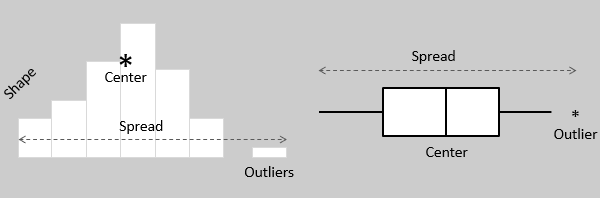Both histograms and box plots are used to explore and present the data in an easy and understandable manner. Histograms are preferred to determine the underlying probability distribution of a data. Box plots on the other hand are more useful when comparing between several data sets. They are less detailed than histograms and take up less space.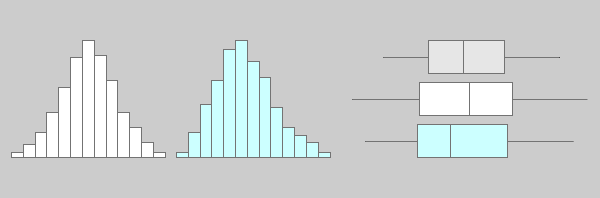Although histograms are better in displaying the distribution of data, you can use a box plot to tell if the distribution is symmetric or skewed. In a symmetric distribution, the mean and median are nearly the same, and the two whiskers has almost the same length.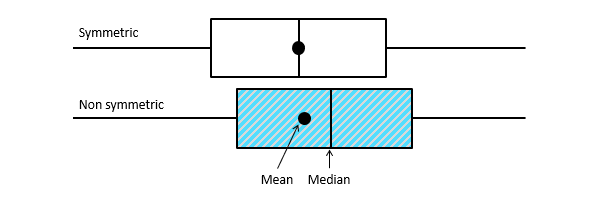You can use histograms and box plots to verify whether an improvement has been achieved by exploring the data before and after the improvement initiative. Both tools can be helpful to identify whether variability is within specification limits, whether the process is capable, and whether there is a shift in the process over time.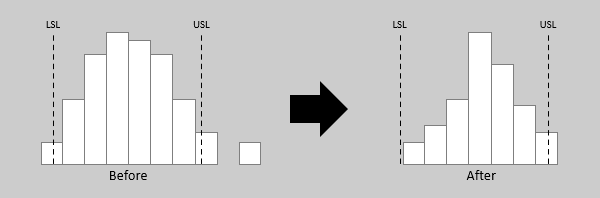Both histograms and box plots are ideal to represent moderate to large amount of data. They may not accurately display the distribution shape if the data size is too small. In practice, a sample size of at least 30 data values would be sufficient for both tools.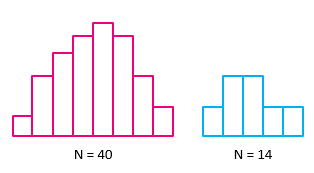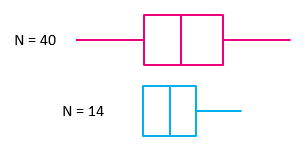Many statistical applications allow the option of summarizing your data graphically (including plotting the data on histograms and box plots as shown below). This can reveal unusual observations in your data that should be investigated before performing detailed statistical analysis.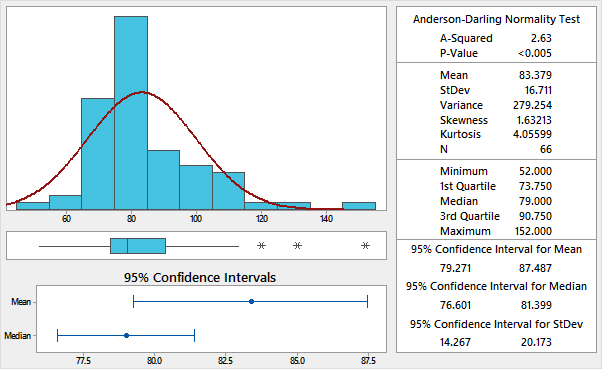## Conclusion:

Histograms and box plots are very similar in that they both help to visualize and describe numeric data. Although histograms are better in determining the underlying distribution of the data, box plots allow you to compare multiple data sets better than histograms as they are less detailed and take up less space. It is recommended that you plot your data graphically before proceeding with further statistical analysis.

Related Articles